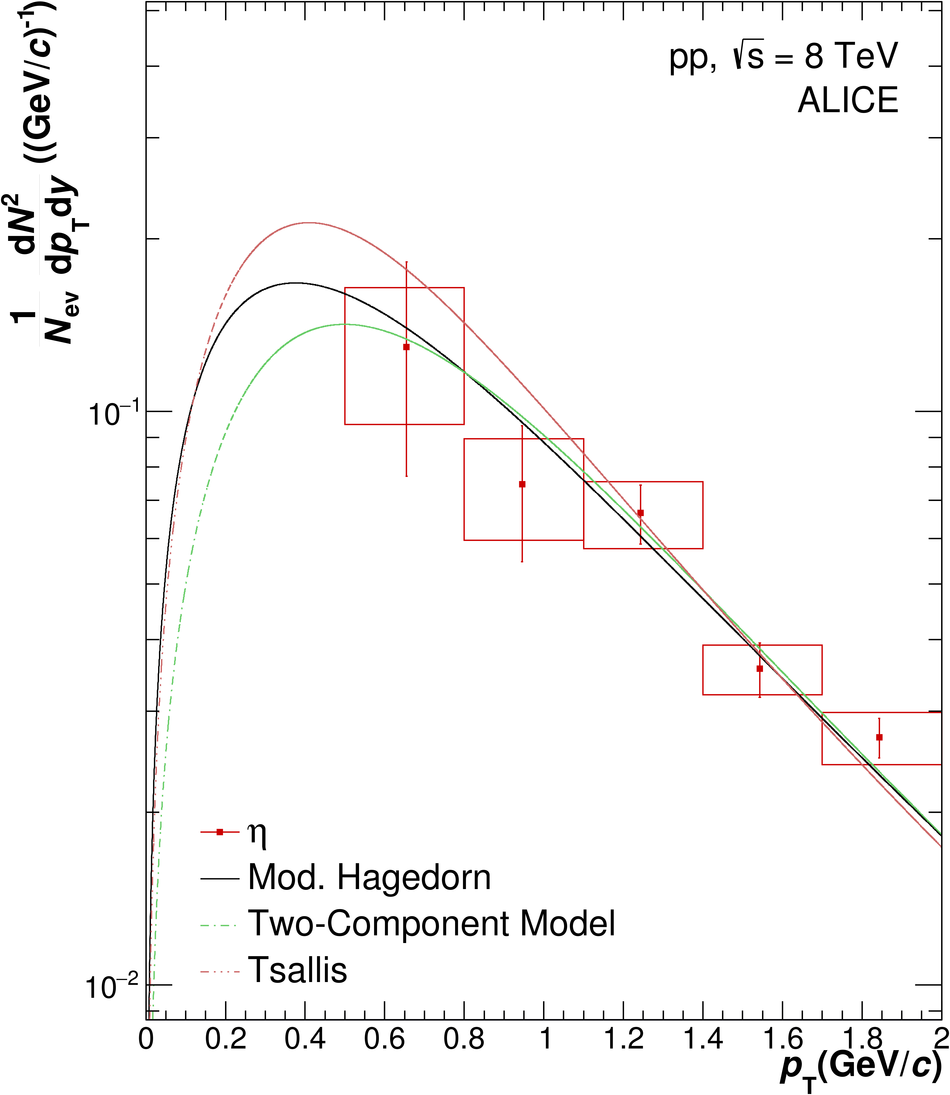# $π^0$ and $η$ meson production in proton-proton collisions at $\sqrt{s}=8$ TeV

An invariant differential cross section measurement of inclusive $\pi^{0}$ and $\eta$ meson production at mid-rapidity in pp collisions at $\sqrt{s}=8$ TeV was carried out by the ALICE experiment at the LHC. The spectra of $\pi^{0}$ and $\eta$ mesons were measured in transverse momentum ranges of $0.3<~p_{\rm T}<~35$ GeV/$c$ and $0.5<~p_{\rm T}<~35$ GeV/$c$, respectively. Next-to-leading order perturbative QCD calculations using fragmentation functions DSS14 for the $\pi^{0}$ and AESSS for the $\eta$ overestimate the cross sections of both neutral mesons, although such calculations agree with the measured $\eta/\pi^{0}$ ratio within uncertainties. The results were also compared with PYTHIA~8.2 predictions for which the Monash~2013 tune yields the best agreement with the measured neutral meson spectra. The measurements confirm a universal behavior of the $\eta/\pi^{0}$ ratio seen for NA27, PHENIX and ALICE data for pp collisions from $\sqrt{s}=27.5$ GeV to $\sqrt{s}=8$ TeV within experimental uncertainties. A relation between the $\pi^{0}$ and $\eta$ production cross sections for pp collisions at $\sqrt{s}=8$ TeV is given by $m_{\rm T}$ scaling for $p_{\rm T}>3.5$ GeV/$c$. However, a deviation from this empirical scaling rule is observed for transverse momenta below $p_{\rm T}<~3.5$ GeV/$c$ in the $\eta/\pi^0$ ratio with a significance of $6.2\sigma$.

Figures

## Figure 1

 Determination of $RF$s for the PHOS-L0 and EMC-L0/L1 triggers. In the plateau region, the $RF$s are obtained by fits of constants in the given cluster energy ranges, illustrated by the dotted lines. The uncertainties of the determined $RF$s are indicated by light colored uncertainty bands, which are obtained by varying the fit ranges.## Figure 2

 Example invariant mass spectra in selected $p_{\rm T}$ slices for PCM (top left), PHOS (top right), EMC (bottom left) and PCM-EMC (bottom right) in the $\pi^{0}$ mass region The black histograms show raw invariant mass distributions before any background subtraction The grey points show mixed-event and residual correlated background contributions, which have been subtracted from raw real events to obtain the signal displayed with red data points The blue curves represent fits to the background-subtracted invariant mass spectra Additional examples of invariant mass distributions for the different methods are given in Ref. .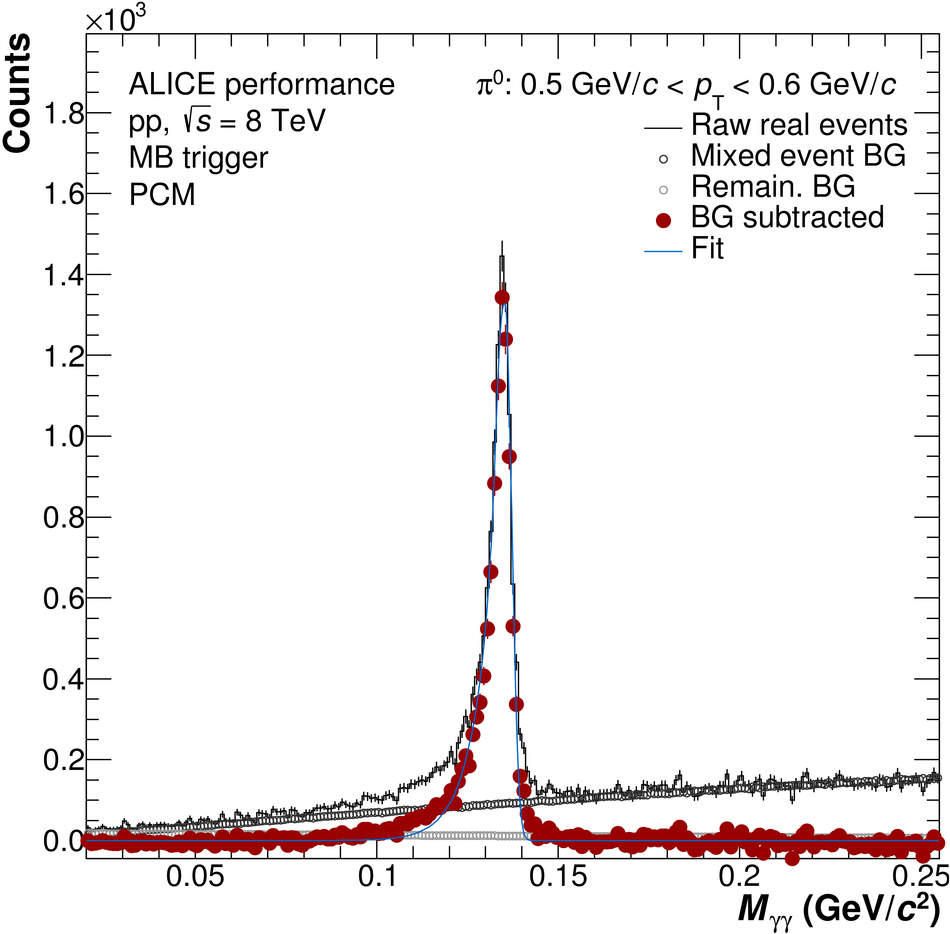## Figure 3

 Example invariant mass spectra in selected \pT slices in PCM (top left), PCM-EMCal (top right) and EMCal (bottom plots) in the $\eta$ mass region The black histograms show raw invariant mass distributions before any background subtraction The grey points show mixed-event and residual correlated background contributions, which have been subtracted from raw real events to obtain the signal displayed with red data points The blue curves represent fits to the background-subtracted invariant mass spectra Additional examples of invariant mass distributions for the different methods are given in Ref. .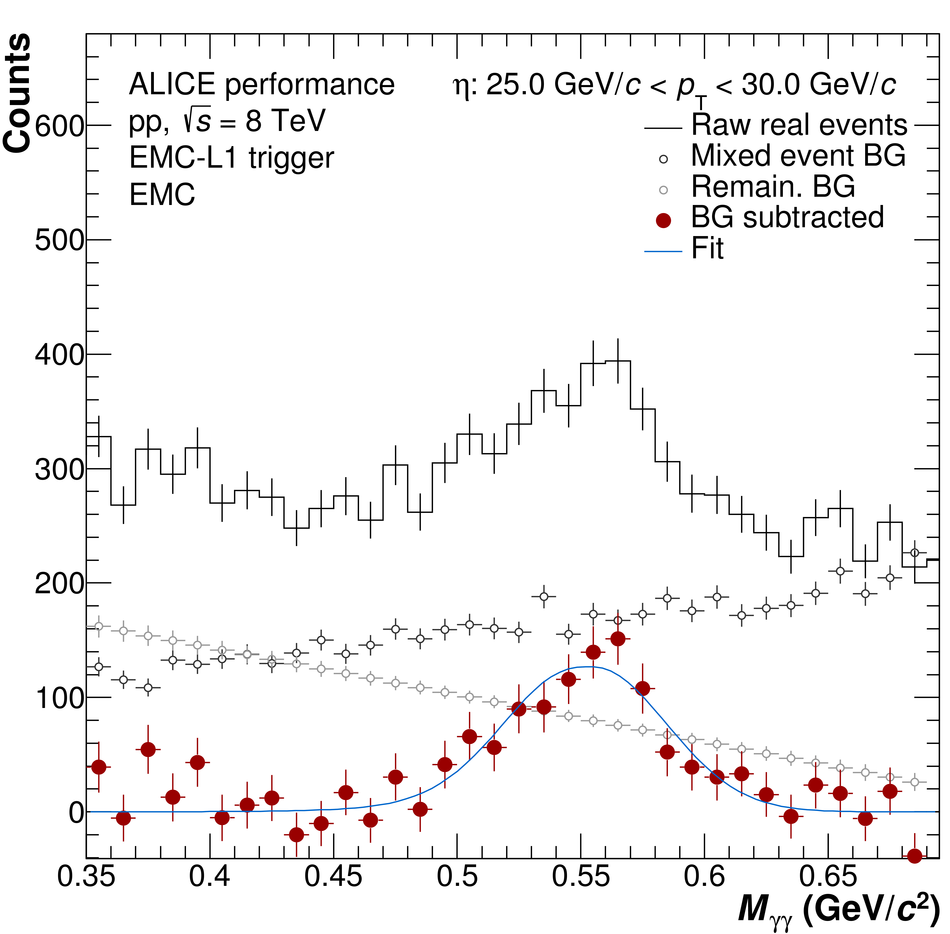## Figure 4

 The left plots show reconstructed $\pi^{0}$ peak positions (left-bottom) and widths (left-top) of each reconstruction method compared to MC simulations for the transverse momentum bins used in the analysis. Corresponding plots for the $\eta$ meson are on the right for peak masses (right-bottom) and widths (right-top).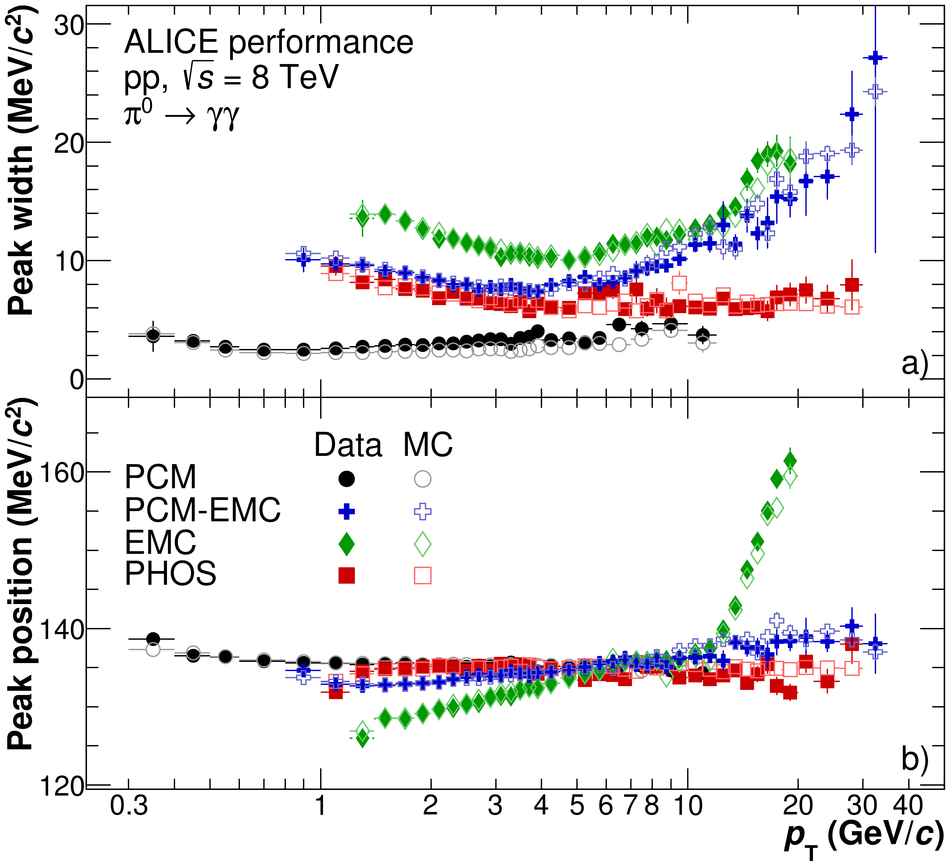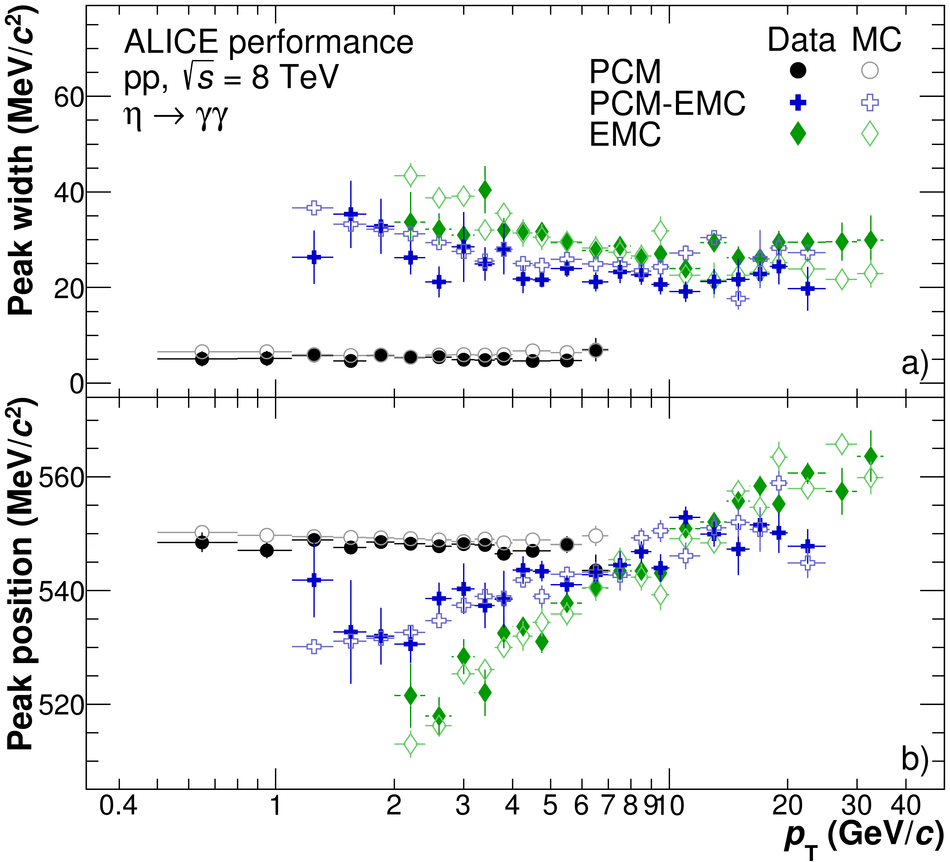## Figure 5

 The normalized correction factors, $\epsilon$, for each reconstruction method for $\pi^{0}$ (left) and $\eta$ mesons (right) plotted versus \pT bins used in the analysis The factors contain the detector acceptances and the respective reconstruction efficiencies, where acceptances are further normalized by the rapidity windows accessible with each method, $\Delta y$, and full azimuth coverage of $2\pi$, in order to enable a direct comparison between the different methods.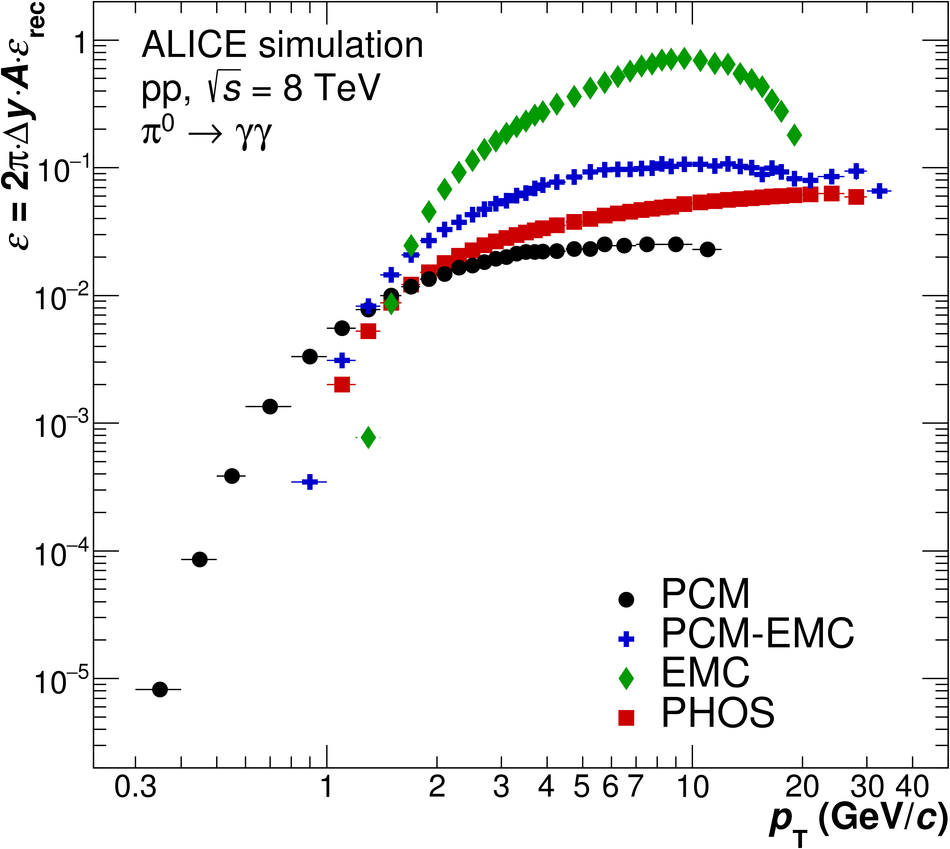## Figure 6

 Ratios of the fully corrected $\pi^{0}$ (left) and $\eta$ (right) spectra for each reconstruction method to the TCM fit of the combined spectrum.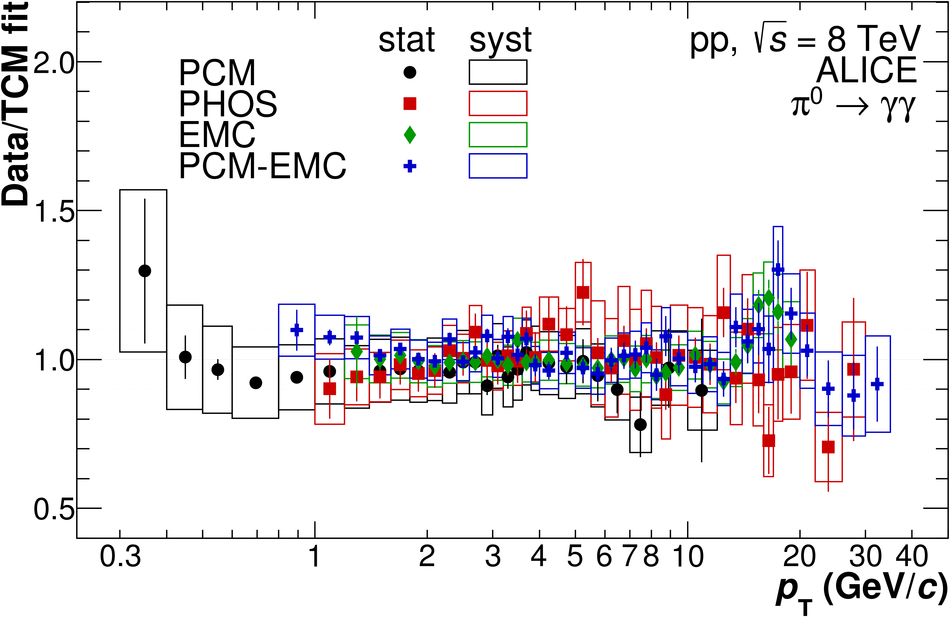## Figure 7

 Invariant cross sections for neutral meson production are shown together with NLO pQCD predictions using PDFs MSTW08 (CTEQ6M5) with FFs DSS14 (AESSS) for $\pi^{0}$ ($\eta$) as well as PYTHIA8.210 calculations, for which two different tunes are available. The data points are fitted using a TCM fit, Eq.~\ref{eq:Bylinkin}, and a Tsallis fit, Eq.~\ref{eq:Tsallis}.## Figure 8

 Left: $\eta/\pi^{0}$ ratio compared to NLO pQCD predictions using PDF CTEQ6M5 and FFs DSS07 for the $\pi^0$ and AESSS for the $\eta$, and PYTHIA8.210 calculations using Tune~4C and Monash~2013 tune. The total uncertainties of the measured $\eta/\pi^{0}$ ratio are of the order of $10$\% for most of the \pT bins covered, increasing for lower and higher momenta due to limited statistics as well as systematic effects. Right: Comparison of the $\eta/\pi^{0}$ ratio to related, previous ALICE measurements as well as other experiments at lower collision energies, for which total uncertainties are drawn. Furthermore, a comparison to the $\eta/\pi^{0}$ ratio obtained with \mT scaling is added.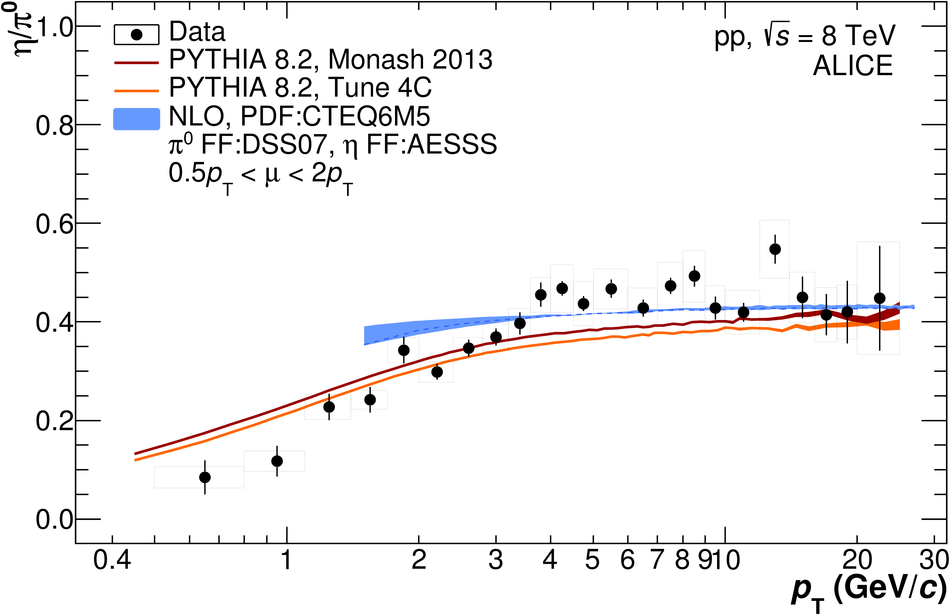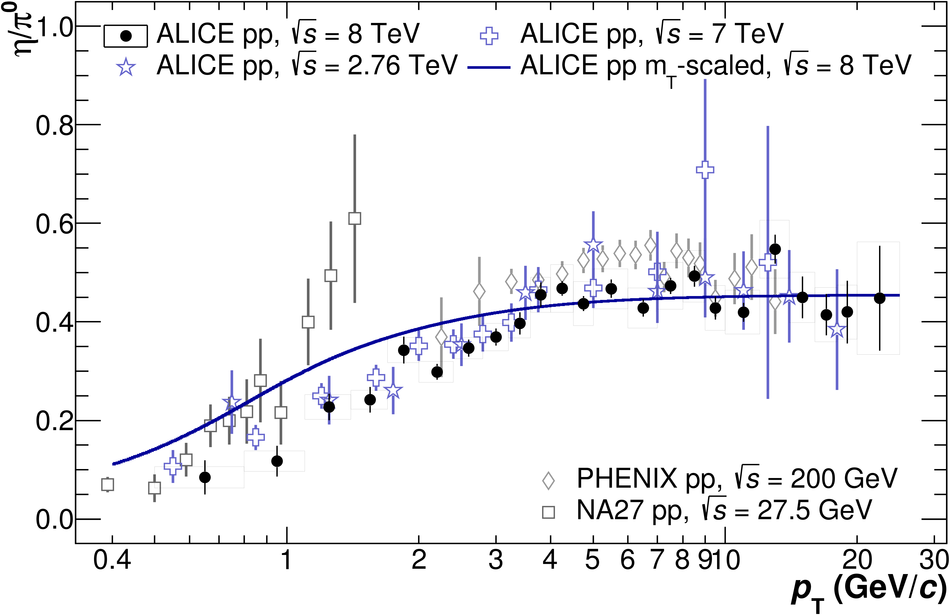## Figure 1

 Example invariant mass distributions in the $\pi^{0}$ peak region for PCM-EMCal (upper left), EMCal (upper right), PHOS (lower left) and PCM (lower right) for the MB trigger.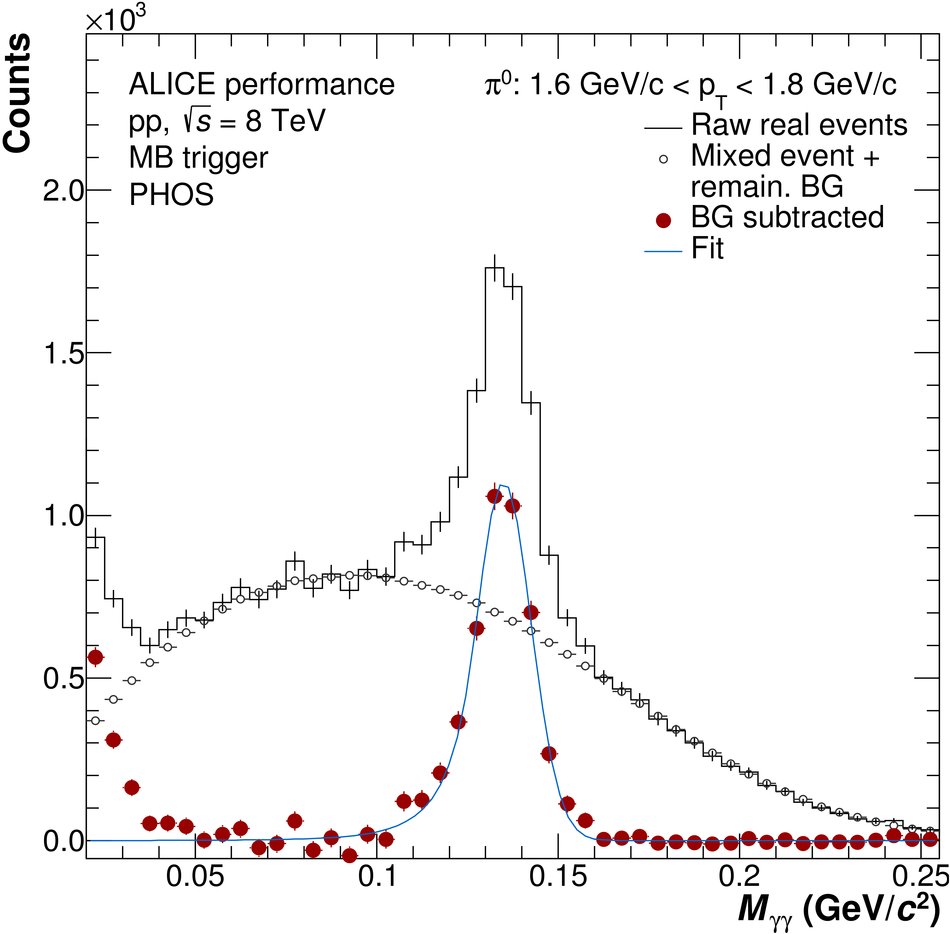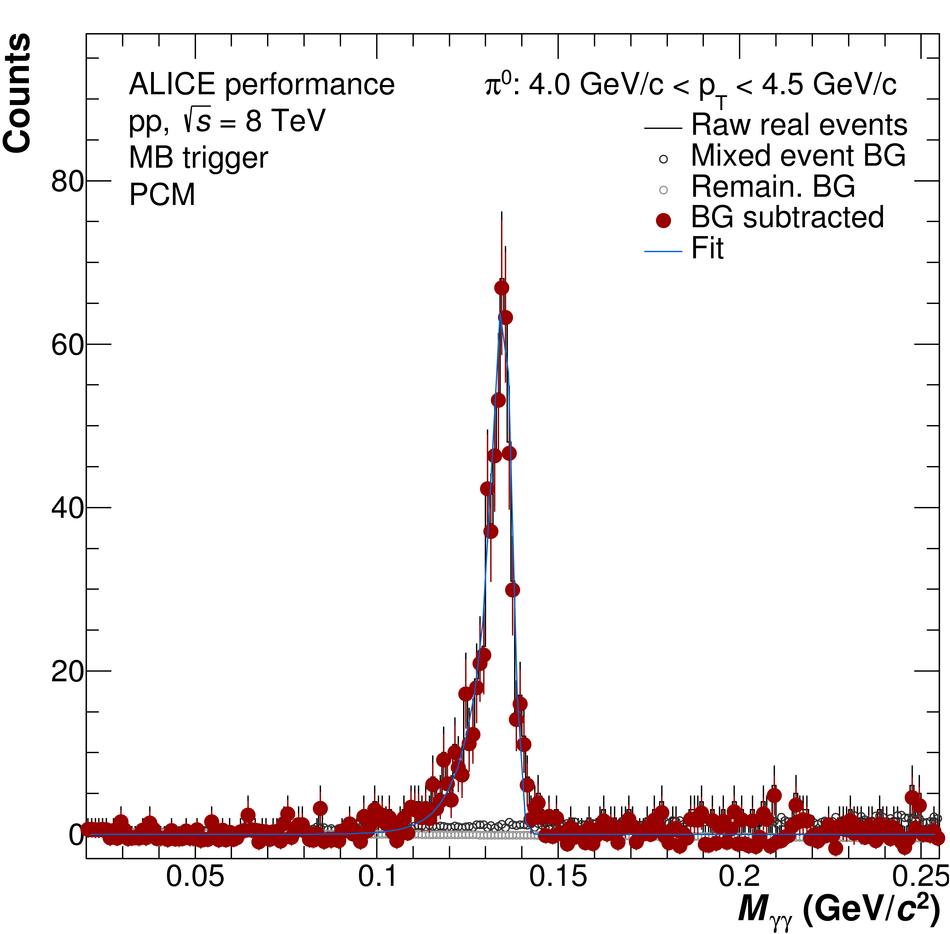## Figure 2

 Example invariant mass distributions in the $\pi^{0}$ peak region for PHOS (upper left) using the MB trigger, EMC-L0 triggered PCM-EMCal (upper right), PHOS (lower left) using PHOS trigger and EMCal (lower right) using the EMC-L1 trigger.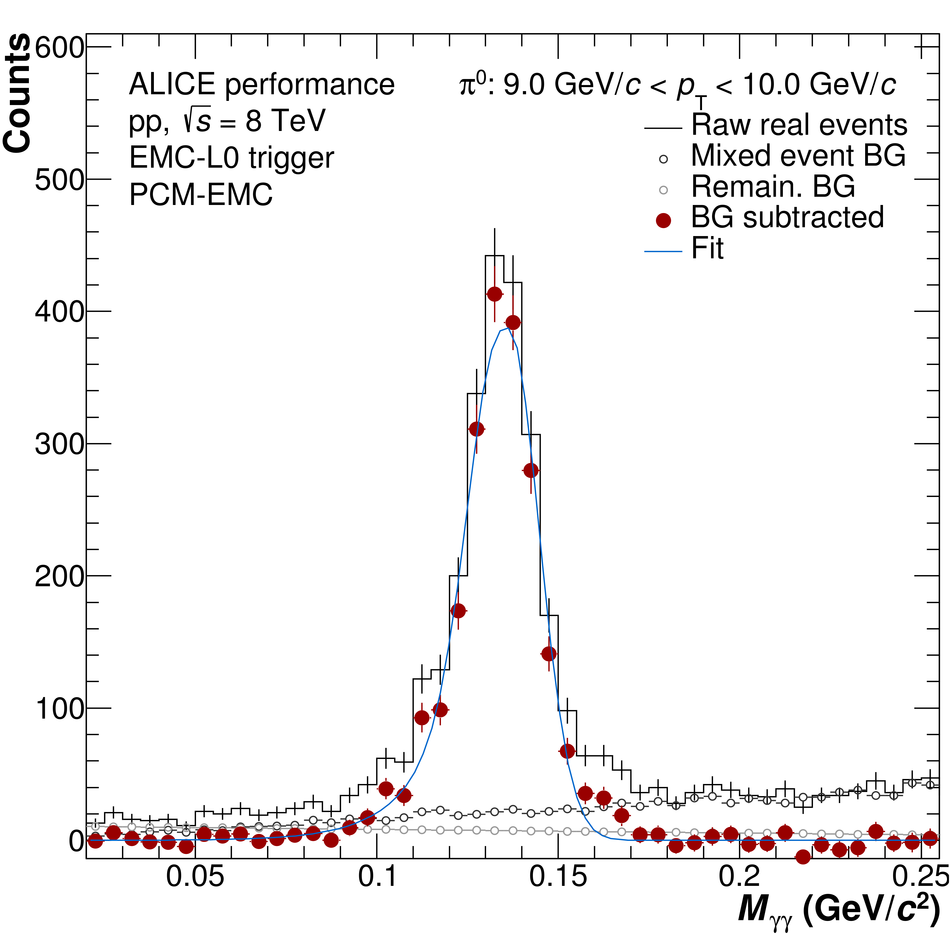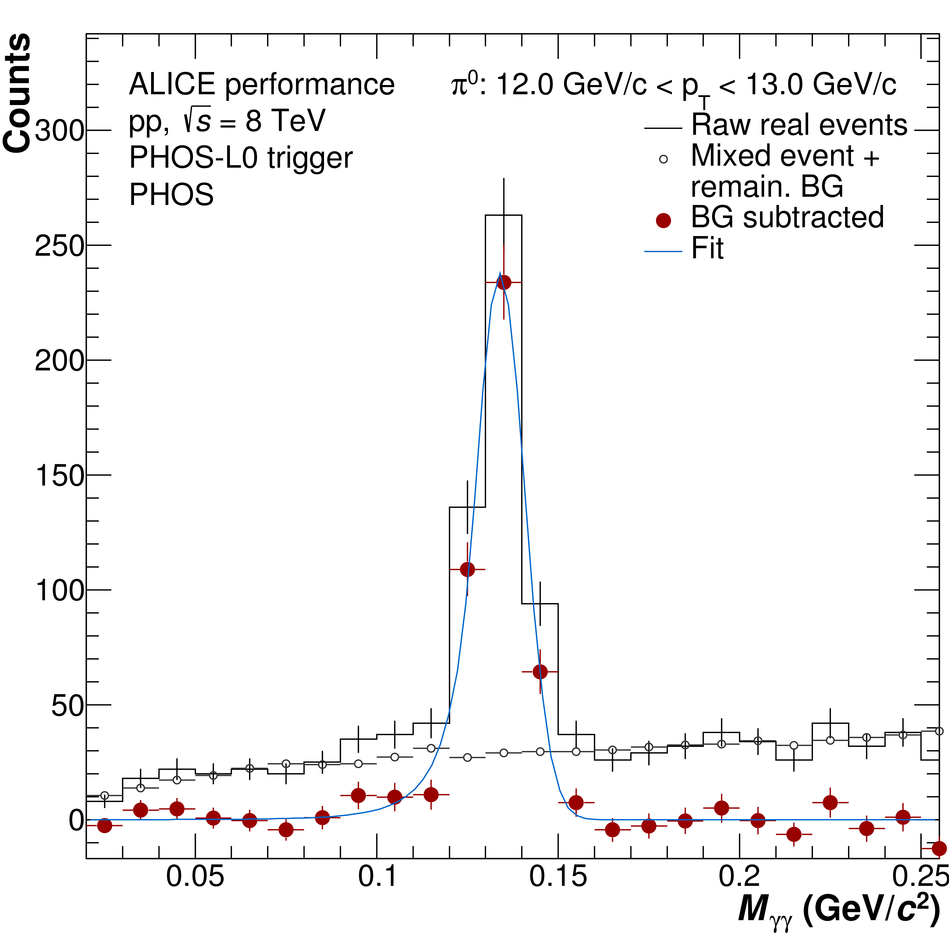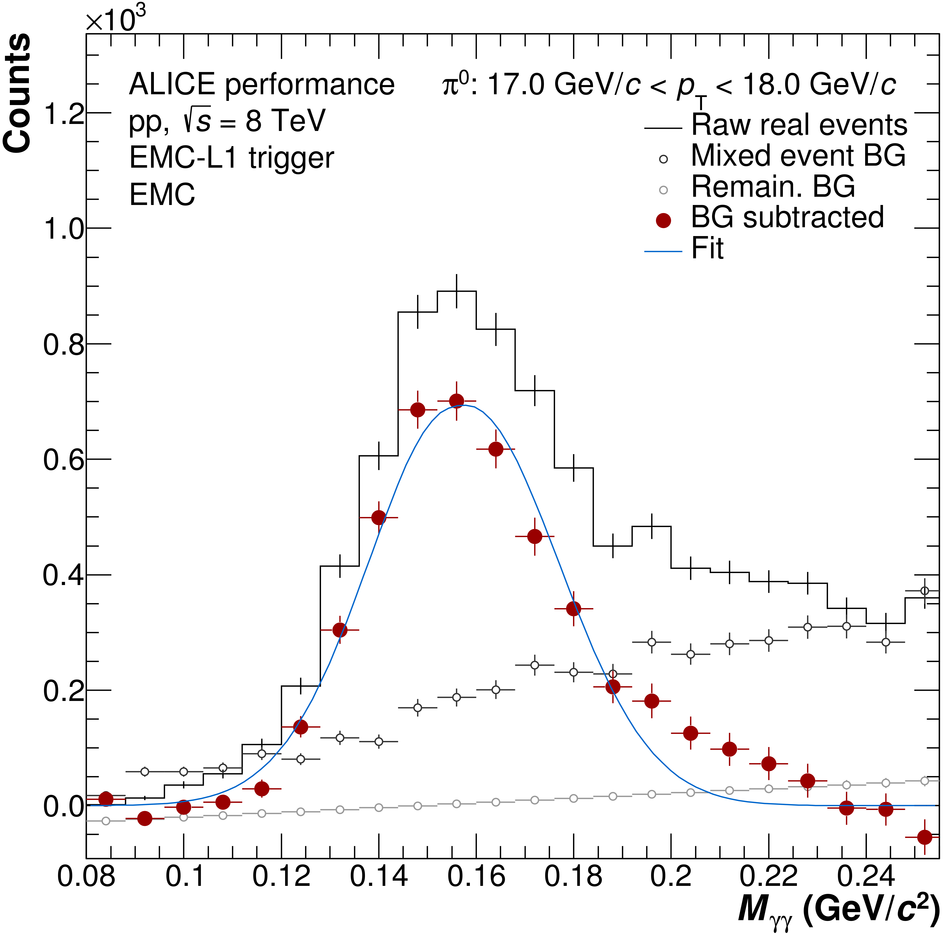## Figure 3

 Example invariant mass distributions in the $\eta$ peak region for EMCal (upper left) and PCM (upper right) using the MB trigger, and PCM-EMCal (lower left and lower right) using the EMC-L0 trigger as well as the EMC-L1 trigger.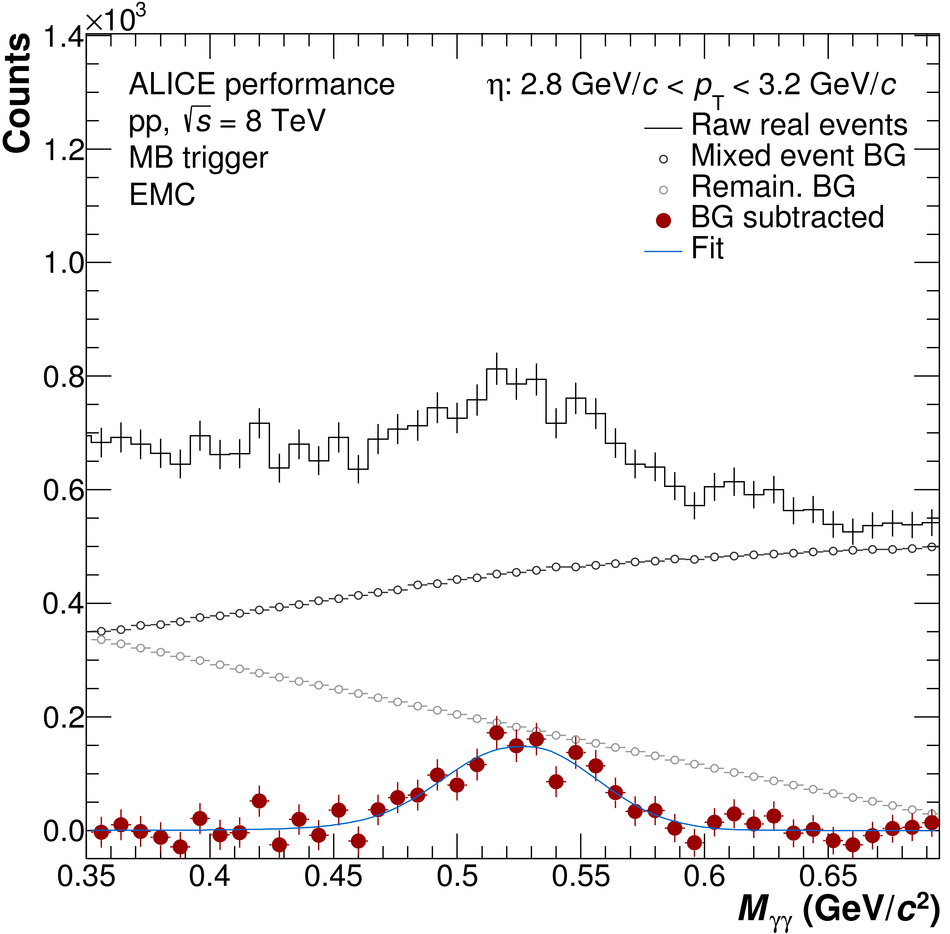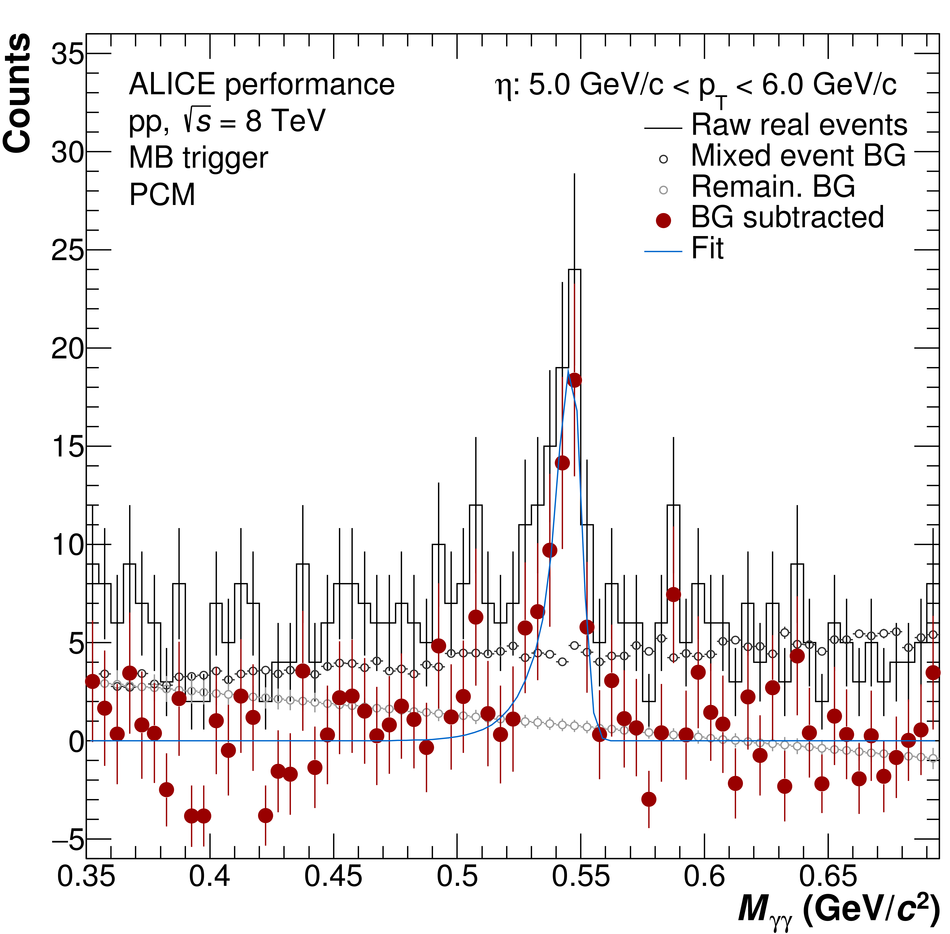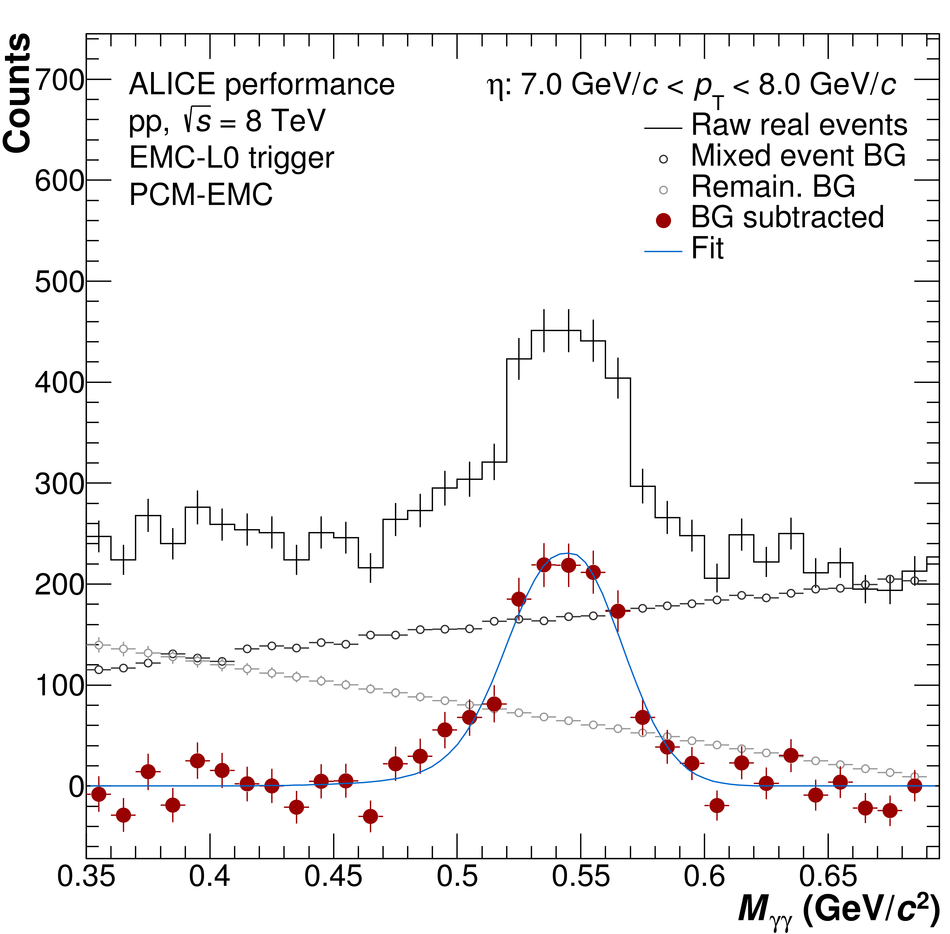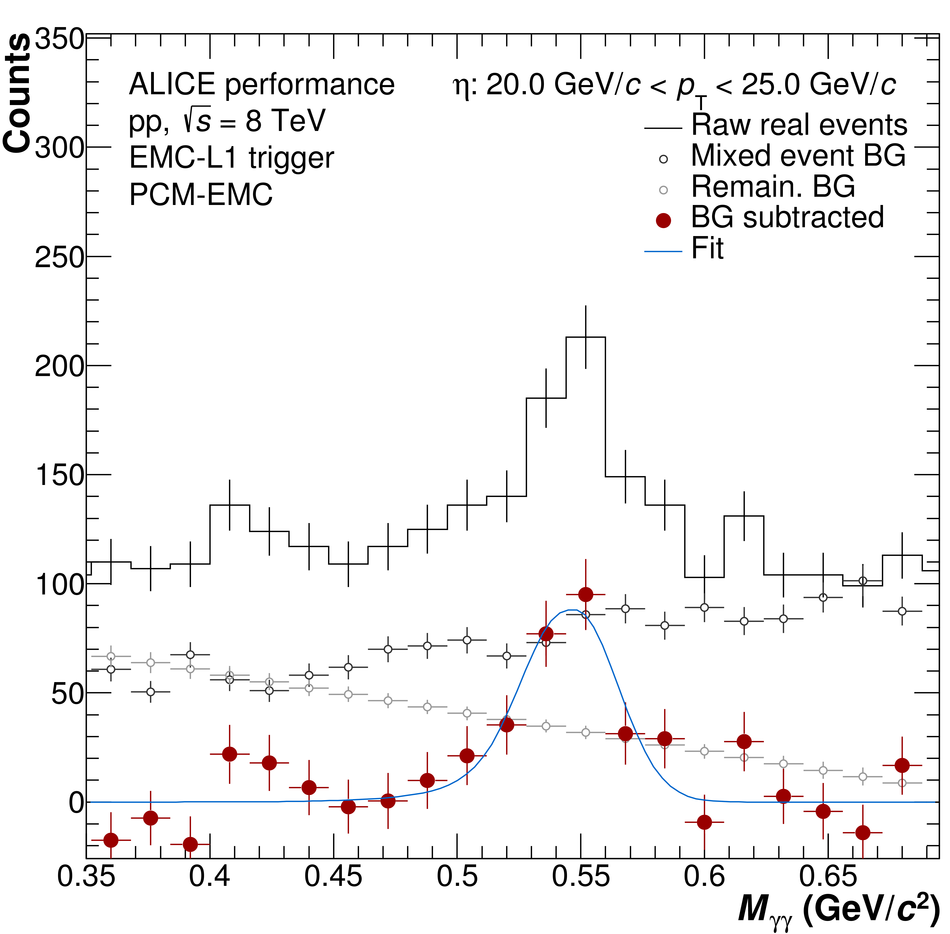## Figure 4

 Invariant differential cross sections of $\pi^{0}$ and $\eta$ mesons for pp collisions at $\sqrt{s}=8$~TeV. The two-component model (TCM) as well as Tsallis fits to the data are also drawn. Furthermore, the data is compared to the PYTHIA~8.210 Tune~4C and the Monash~2013 tune and recent NLO pQCD calculations, for which details on PDF and FF are given in the figure.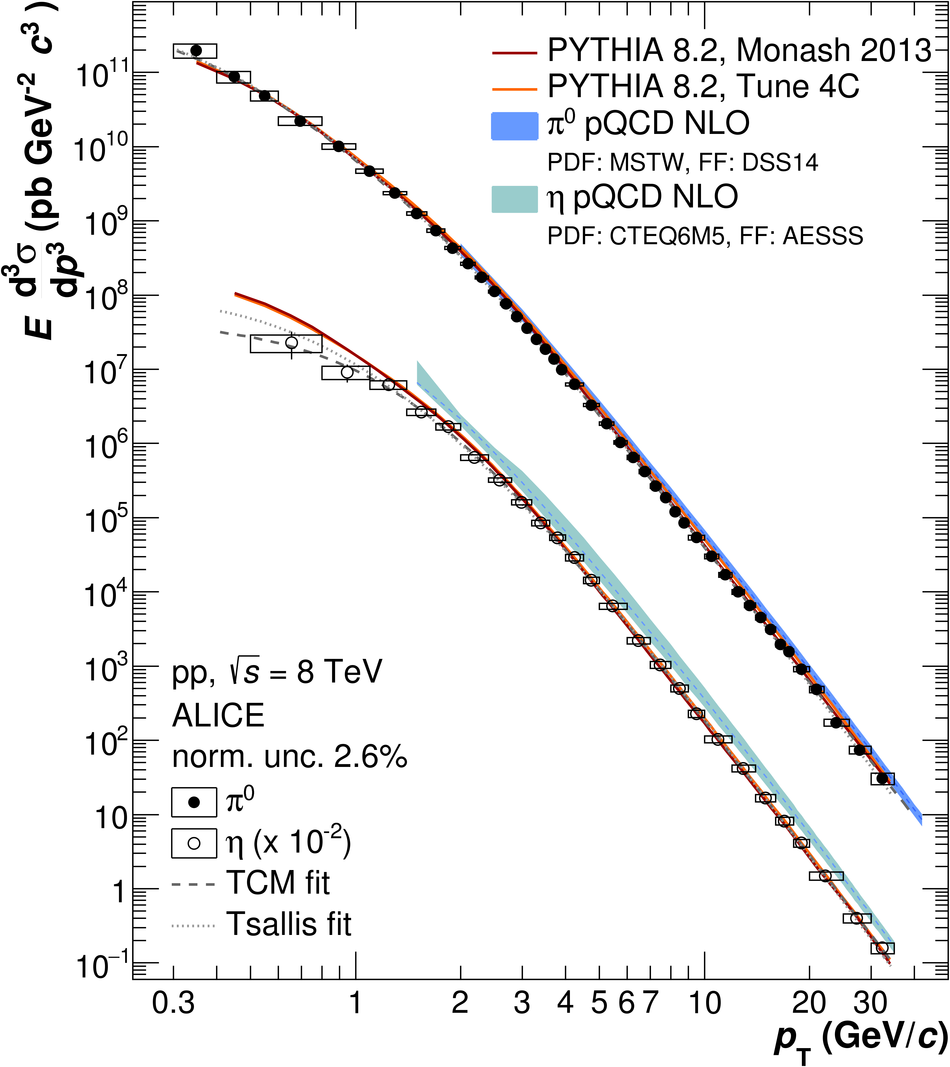## Figure 5

 Ratios of data and theory predictions to the TCM fit of the measured invariant differential cross section of $\pi^{0}$ mesons for pp collisions at $\sqrt{s}=8$~TeV. Besides the data, ratios of theory predictions from PYTHIA~8.210 Tune~4C and Monash~2013 tune as well as of recent NLO pQCD calculations to the TCM fit of the $\pi^{0}$ meson measurement are shown (left). A comparison using some older FF (right) is given as well.## Figure 6

 Invariant differential cross sections of $\pi^{0}$ and $\eta$ mesons for pp collisions at $\sqrt{s}=8$~TeV (left + right). In addition, the right plot contains fits to the data, namely a TCM and a Tsallis fit.## Figure 7

 Ratios of data and theory predictions to the TCM fit of the measured invariant differential cross section of $\eta$ mesons for pp collisions at $\sqrt{s}=8$~TeV. Besides the data, ratios of theory predictions from PYTHIA~8.210 Tune~4C and Monash~2013 tune as well as of recent NLO pQCD calculations to the TCM fit of the $\eta$ meson measurement are shown.## Figure 8

 Ratios of the data to TCM, Tsallis, mod. Hagedorn and power law fits for the neutral pion (left) and the $\eta$ meson (right).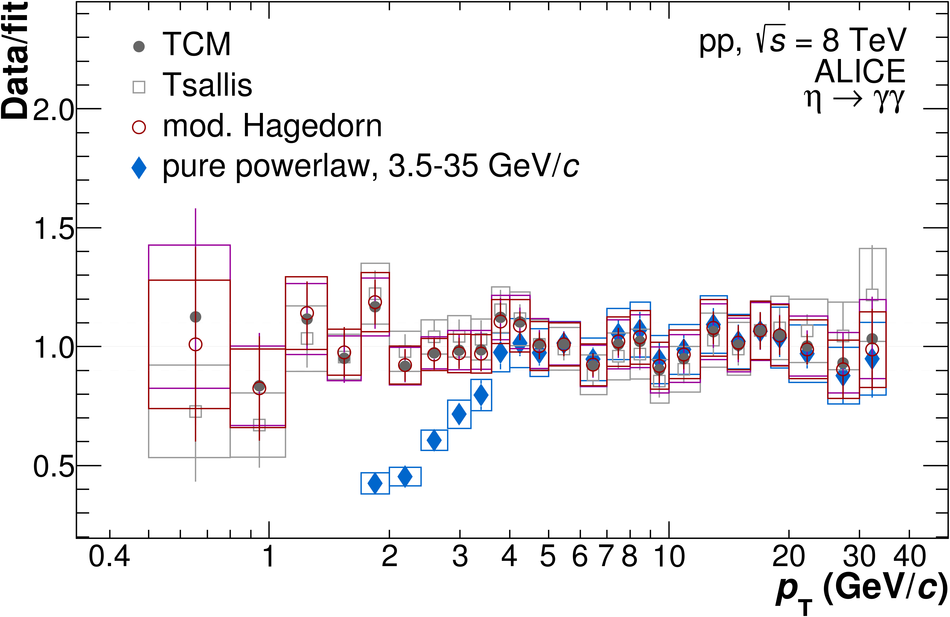## Figure 9

 $\eta/\pi^{0}$ ratio for pp collisions at $\sqrt{s}=8$~TeV in logarithmic (left) and linear scale on x-axis (right).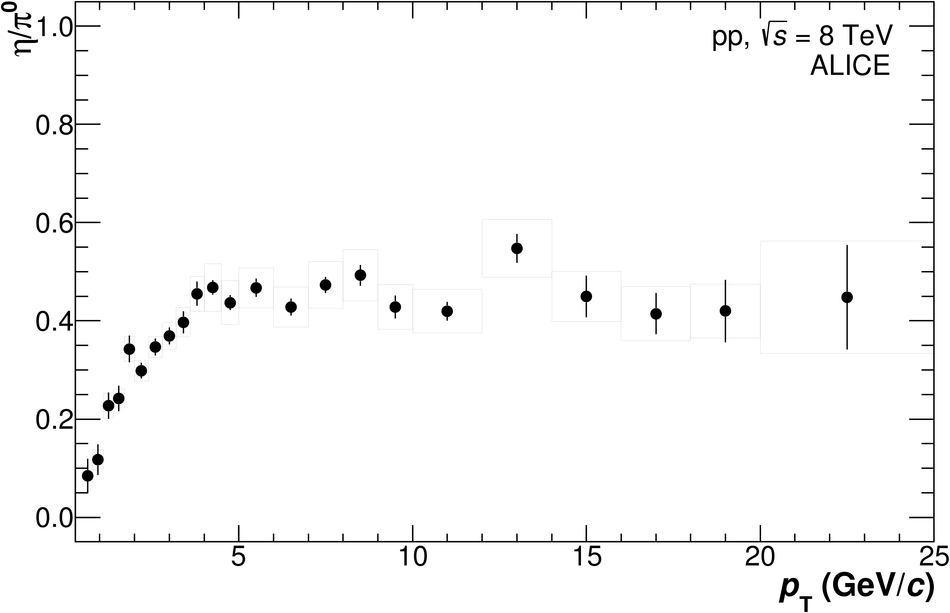## Figure 10

 (left) The measured $\eta/\pi^{0}$ ratio for pp collisions at $\sqrt{s}=8$~TeV is shown together with theory predictions, using a linear scale on x-axis. (right) The plot shows the ratio of the measured $\eta/\pi^0$ ratio over the $\eta/\pi^0$ ratio obtained with $m_{\rm T}$ scaling. Below $3.5~\ensuremath{\mbox{GeV}/c}$, the disagreement from $m_{\rm T}$ scaling hypothesis is found to be $6.2\sigma$.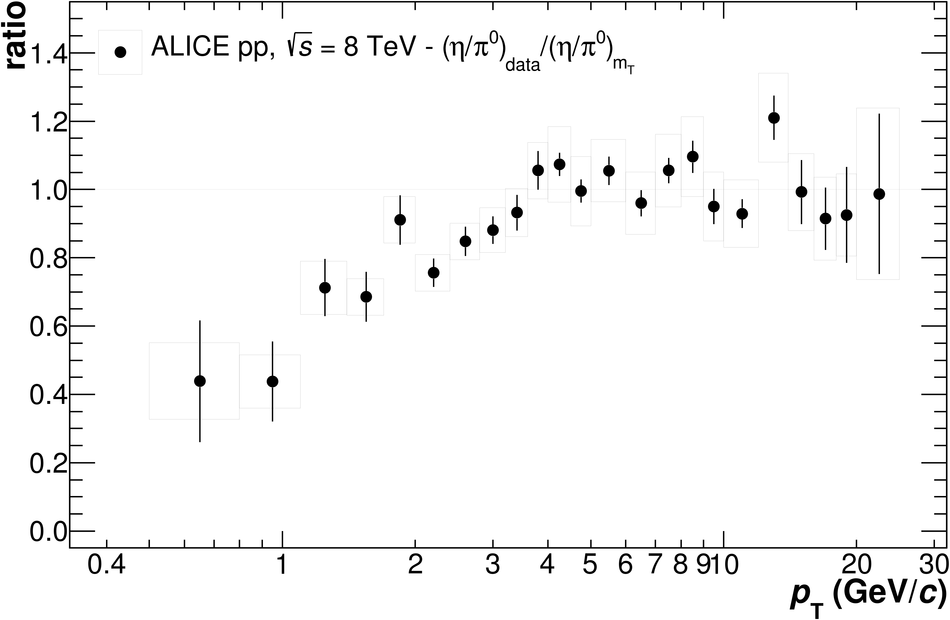## Figure 11

 (left) The integrated yields per inelastic collision, $\text{d}N/\text{d}y$, are plotted for neutral meson measurements in pp collisions at $\sqrt{s}=2.76$ and $8$~TeV. (right) The mean transverse momenta, $\langle \ensuremath{p_{\mbox{\tiny T}}}\rangle$, are plotted for neutral meson measurements in pp collisions at $\sqrt{s}=2.76$ and $8$~TeV. Statistical and systematic uncertainties are given in both plots as well as the systematic uncertainty due to the choice of the fitting function .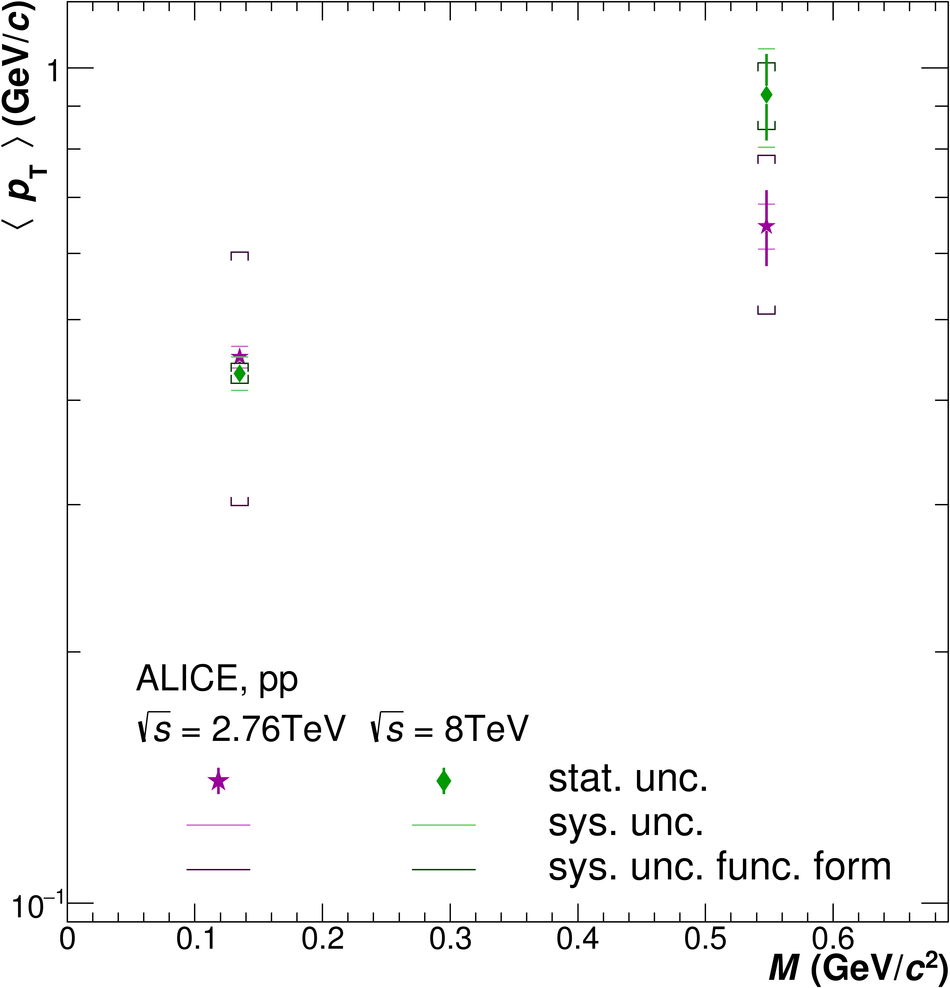## Figure 12

 (left) Differential yield per inelastic pp collision for the neutral pion measurement at $\sqrt{s}=8$~TeV with logarithmic x-axis and (right) showing only the low momentum region $< 2$~\ensuremath{\mbox{GeV}/c} with linear scale on x-axis. The different fits as described in the previous paragraph are shown which are used to determine the integrated yields and mean transverse momenta.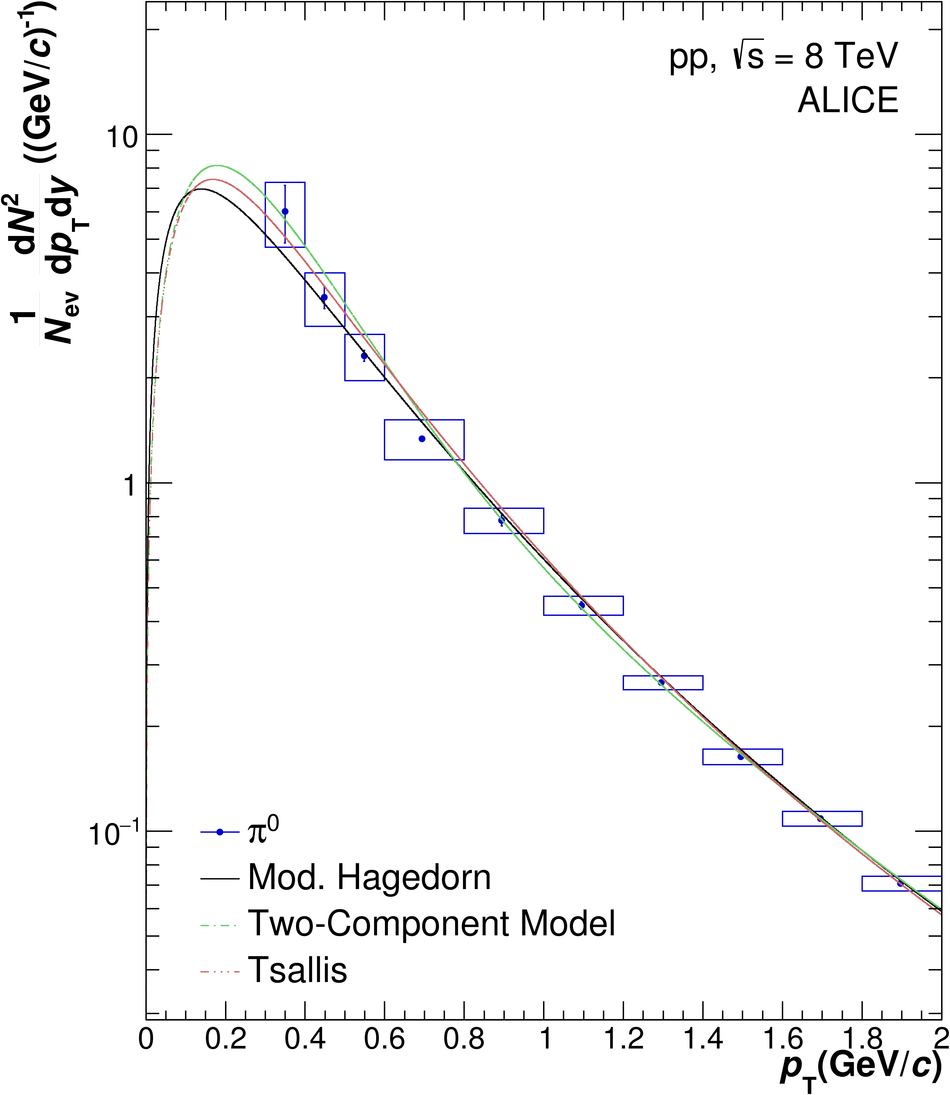## Figure 13

 (left) Differential yield per inelastic pp collision for the $\eta$ meson measurement at $\sqrt{s}=8$~TeV with logarithmic x-axis and (right) showing only the low momentum region $< 2$~\ensuremath{\mbox{GeV}/c} with linear scale on x-axis. The different fits as described in the previous paragraph are shown which are used to determine the integrated yields and mean transverse momenta.